# Application Of Overlay Modeling And Control With Zernike Polynomials In An HVM Environment

How to reduce co-linearity and improve overlay stability.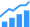By JawWuk Ju, MinGyu Kim and JuHan Lee of SK Hynix; Jeremy Nabeth, John C. Robinson and Bill Pierson of KLA-Tencor; and Sanghuck Jeon and Hoyoung Heo of KLA-Tencor Korea.

Abstract
Shrinking technology nodes and smaller process margins require improved photolithography overlay control. Generally, overlay measurement results are modeled with Cartesian polynomial functions for both intra-field and inter-field models and the model coefficients are sent to an advanced process control (APC) system operating in an XY Cartesian basis. Dampened overlay corrections, typically via exponentially or linearly weighted moving average in time, are then retrieved from the APC system to apply on the scanner in XY Cartesian form for subsequent lot exposure. The goal of the above method is to process lots with corrections that target the least possible overlay misregistration in steady state as well as in change point situations. In this study, we model overlay errors on product using Zernike polynomials with same fitting capability as the process of reference (POR) to represent the wafer-level terms, and use the standard Cartesian polynomials to represent the field-level terms. APC calculations for wafer-level correction are performed in Zernike basis while field-level calculations use standard XY Cartesian basis. Finally, weighted wafer-level correction terms are converted to XY Cartesian space in order to be applied on the scanner, along with field-level corrections, for future wafer exposures. Since Zernike polynomials have the property of being orthogonal in the unit disk we are able to reduce the amount of collinearity between terms and improve overlay stability. Our real time Zernike modeling and feedback evaluation was performed on a 20-lot dataset in a high volume manufacturing (HVM) environment. The measured on-product results were compared to POR and showed a 7% reduction in overlay variation including a 22% reduction in linear model terms variation. This led to an on-product raw overlay Mean + 3Sigma X&Y improvement of 5% and resulted in 0.1% yield improvement.

1. INTRODUCTION
For many process nodes, polynomial functions have been used to model overlay errors across the wafer and field for semiconductor manufacturing process control. In the past, these models were linear in the wafer and field coordinates, and the relatively small numbers of adjustable parameters were largely orthogonal. As the industry has advanced to smaller process nodes, however, a significant increase in the number of adjustable parameters (correctables) has increased significantly. Increased sampling and high order modeling has been adopted in to order model the increased number of knobs required for process control at advanced nodes. With high-order non-linear modeling, comes interaction and correlation between model terms. There are several techniques used to decrease correlation between terms including advanced sampling, modeling algorithms, and APC techniques. In this study we investigate a different parameterization of the overlay models to reduce co-linearity.

Zernike and Legendre polynomials are different parameterizations of regular XY Cartesian polynomials commonly used to model overlay, based on combinations of standard terms. Zernike polynomials are orthogonal on the unit disc, making them suitable for wafer level modeling when normalized to the wafer diameter. The goal of this parameterization is to reduce cross-talk between terms.

In this paper, we evaluate the application of Zernike modeling  and control for wafer-level overlay in an HVM environment. First, we describe the setup for on-product evaluation of Zernike modeling and explain how the conversion process is performed starting from overlay measurements in XY basis to the APC controller in Zernike basis and back to XY basis in order to apply weighted corrections on the scanner machine for subsequent lots. Second, we explain how the overlay model terms are normalized for the stability metric calculation. Finally, we review the on-product evaluation results starting with statistical metrics of the raw overlay results, then comparing the stability metric and probability density functions of the collinear terms between POR and Zernike control.

2. AUTOMATION SETUP FOR ZERNIKE MODELING AND CONTROL
The conceptual diagram in Figure 1 highlights the five essential steps to allow Zernike modeling and control in an HVM environment. First, the overlay is measured on an inline wafer with a sampling plan that provides accurate representation of the full map data while minimizing the throughput cost. Second, the measurement results are fed into a modeling software such as K-T Analyzer® to calculate the Zernike model terms that best fit the raw overlay data. For this evaluation, a third-order Zernike model was used for wafer-level terms while the field-level overlay data was modeled using the standard intrafield Higher Order Process Corrections (iHOPC) in XY basis . Third, the correction terms are sent to the customer APC system in order to calculate the dampened corrections, typically via exponentially or linearly weighted moving average in time. At this stage, the weighted corrections are in two different basis: XY for intrafield terms and Zernike for interfield terms, hence, in step 4, the interfield terms are converted back into the XY basis (Figure 2). All weighted correction terms in XY basis are then sent to the scanner to be used for subsequent wafer exposure, from the same APC thread.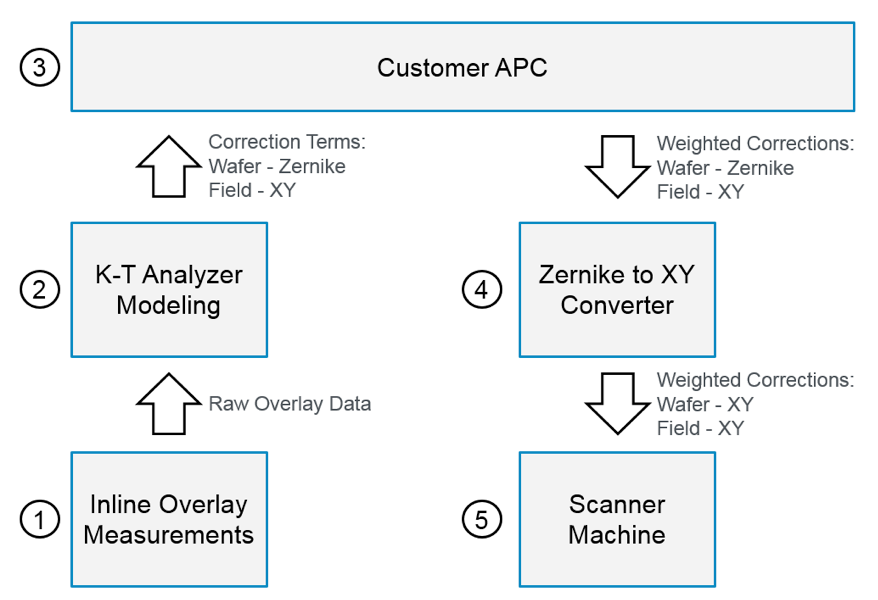Fig. 1: Zernike Modeling and Control Setup.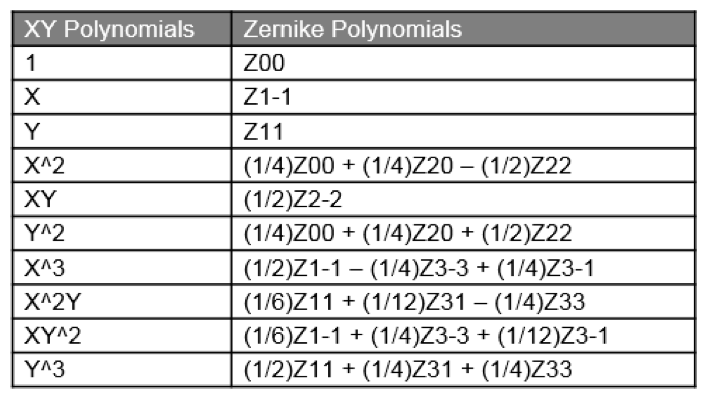Figure 2: Conversion Table from Zernike to XY.

3. NORMALIZATION OF MODELING TERMS AND STABILITY METRIC
In order to evaluate the stability improvement of Zernike modeling and control over the process of reference (POR), we developed a stability metric that requires the normalization of each overlay term by the magnitude of its overlay impact. This is done, in K-T Analyzer®, by individually setting each term to a value of “1” and applying the corresponding process correction on a zero-overlay wafer map with POR layout (Figure 3). We then calculate the root mean square of the overlay results on the wafer map to obtain the normalization multiplier for each term.

The stability of each term is then calculated by taking the standard deviation of the normalized term across all lots and the stability metric is defined as the root sum squared of the stability, for the terms of interest.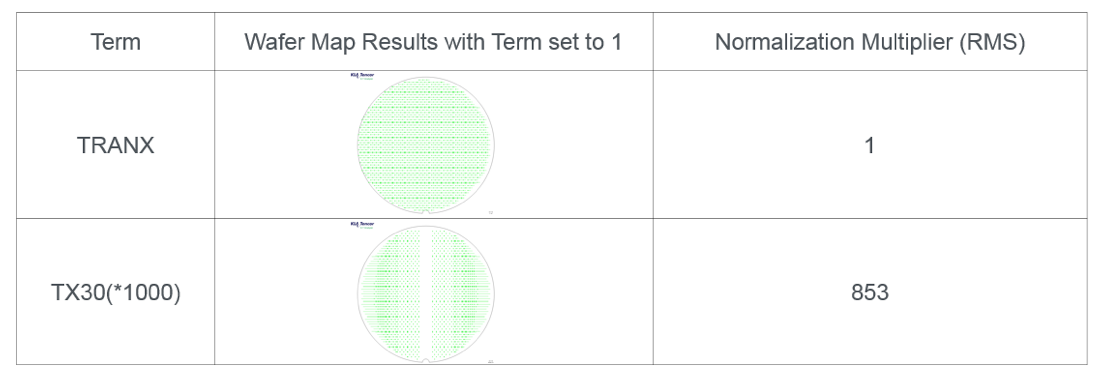Figure 3: Terms Normalization on Arbitrary Full Map Layout.

4. ON-PRODUCT evALUATION RESULTS
In our HVM evaluation, Zernike modeling and control was applied on 100 lots (205 data points) and the performance was compared against 427 lots (854 data points) which used POR modeling and control in XY basis. To ensure that the results were not skewed due to higher order modeling, our Zernike model was set to use the same order as the POR model. By using Zernike, raw overlay |Mean|+3*Sigma (Figure 4) was improved by 5% in X and 7% in Y, which resulted in a yield improvement of 0.11%. The statistical significance of the difference between populations’ means was confirmed by computing the t-test on a 95% confidence interval (Figure 5).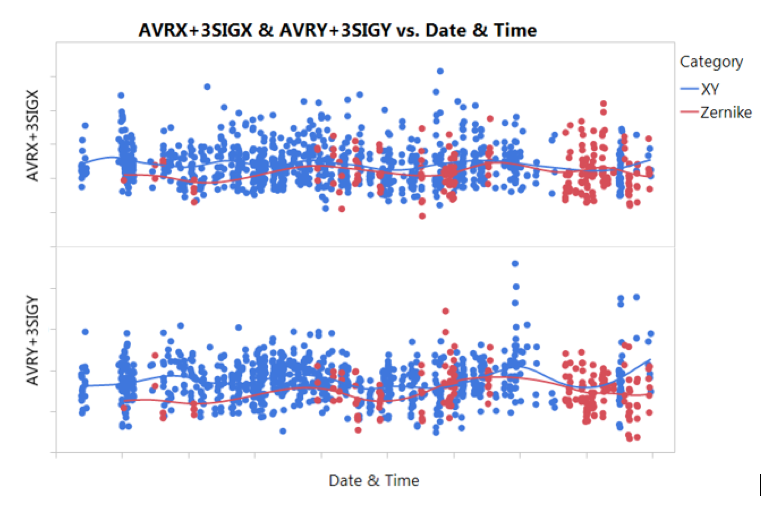Figure 4: Raw Overlay Statistical Results.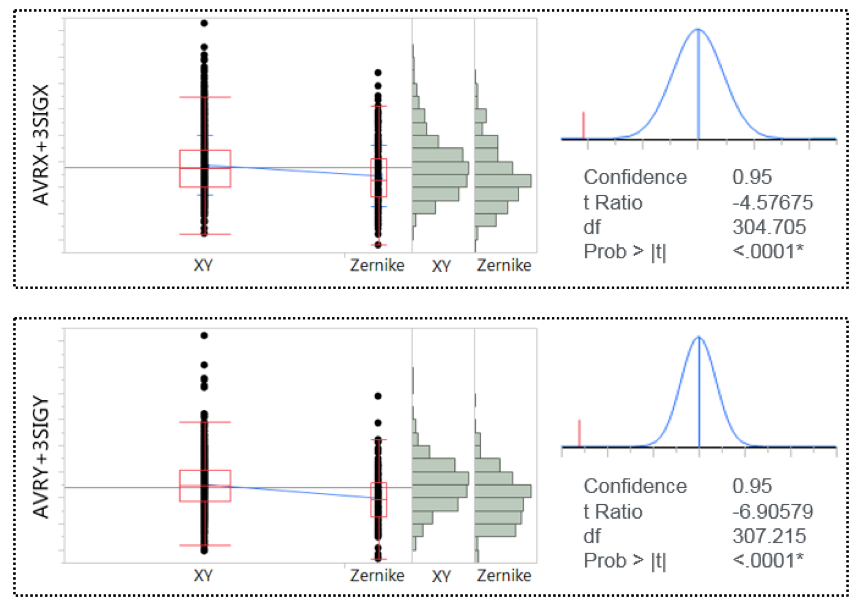Figure 5: |Mean|+3*Sigma t-test Results

The stability metric was calculated on terms with multicollinearity issue: first order vs. third order expansion in X and Y direction. The results in Table 1 show an improvement of 9% when using Zernike modeling and control over XY.

The stability metric was calculated on terms with multicollinearity issue: first order vs. third order expansion in X and Y direction. The results in Table 1 show an improvement of 9% when using Zernike modeling and control over XY.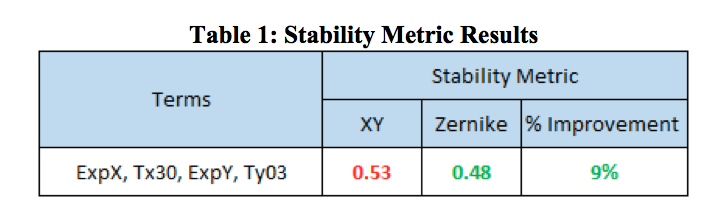The probability density functions of the multicollinear overlay terms were calculated by assuming a Johnson distribution and the Shapiro-Wilk W Test results confirms that this assumption cannot be rejected. Figure 6 shows that the distribution of the first order expansion terms is tighter with Zernike that with POR while the third order distributions appear to be comparable between the two control methods.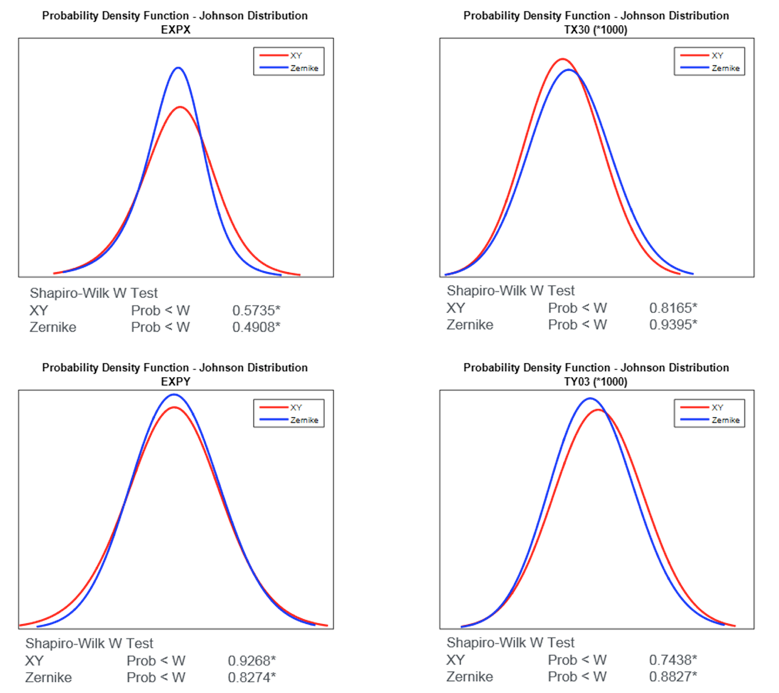Figure 6: Probability Density Functions.

5. SUMMARY
We have evaluated Zernike modeling and control in an HVM environment. The on-product overlay was improved by 5% in X and 7% in Y for |Mean|+3*Sigma which led to a 0.11% yield improvement. A newly defined stability metric was used to quantify the run-to-run stability improvement and showed that multicollinear terms stability was improved by 9%. Lastly, the probability density functions of overlay model terms showed noticeable improvement with Zernike for first order interfield expansion X&Y while the third order expansion appears to be comparable with XY modeling and control. Future work includes the incorporation of Legendre polynomials, as their orthogonality on the unit square make them suitable for field level modeling when normalized to the field size.

References
1. Ju, J., Kim, M., Lee, J., Sherwin, S., Hoo, G., Choi, D., Lee, D., Jeon, S., Lee, K., Tien, D., Pierson, B., Robinson, J., Levy, A., Smith, M., “High order overlay modeling and APC simulation with Zernike-Legendre polynomials”, Proc. SPIE 9424, 94241Y (2015).
2. Huang, C., Chue, C., Liu, A., Wu, W., Shih, C., Chiou, T., Lee, J., Chen, O., Chen, A., “Using Intra-Field High Order Correction to Achieve Overlay Requirement beyond Sub-40nm Node”, Proc. SPIE 7272, 72720I (2009).

This paper was originally published as part of SPIE proceedings March 18, 2016.